Courses

# Previous year Questions (2016-20) - Modern Physics (Part - 2) Notes | EduRev

## JEE : Previous year Questions (2016-20) - Modern Physics (Part - 2) Notes | EduRev

The document Previous year Questions (2016-20) - Modern Physics (Part - 2) Notes | EduRev is a part of the JEE Course Class 12 Physics 35 Years JEE Mains &Advance Past year Paper.
All you need of JEE at this link: JEE

Q 31. A solution containing active cobalt 60/27 Co having activity of 0.8 µCi and decay constant λ is injected in an animal's body. If 1 cm3 of blood is drawn from the animal's body after 10 hrs of injection, the activity found was 300 decays per minute. What is the volume of blood that is flowing in the body? (1 Ci = 3.7 x 1010 decays per second and at t = 10 hrs e–λt = 0.84)     
(1) 4 liters
(2) 5 liters
(3) 6 liters
(4) 7 liters
Ans:
(2)
Solution:
(dn/dt) = −λNo
0.8 µ Ci = −λNo
N1 = No(0.84)
v → N1
v cm3 → N1/v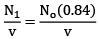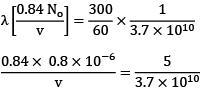v = 3.7/5 × 0.84 × 0.84 × 104 cm3
= 0.5 × 104 cm3 = 5 × 10cm3 = 5 lit

Q 32. The energy required to remove the electron from a singly ionized Helium atom is 2.2 times the energy required to remove an electron from Helium atom. The total energy required to ionize the Helium atom completely is:      
(1) 109 eV
(2) 79 eV
(3) 20 eV
(4) 34 eV
(3)
Solution:
E1 = ionization energy of ionized He
E2 = 2. 2E2
E1 = 13.6 eV
E2 = 13.6/2.2 = 6.18 eV
Total = E1 + E2 = 13.6 ev + 6.18 eV
ETotal = 20 eV

Q 33. At some instant a radioactive sample S1 having an activity 5 μCi has twice the number of nuclei as another sample S2 which has an activity of 10 μCi. The half lives of S1 and S2 are:     
(1) 5 years and 20 years, respectively
(2) 20 years and 5 years, respectively
(3) 20 years and 10 years, respectively
(4) 10 years and 20 years, respectively
Ans:
A
Solution:
Given : N1 = 2N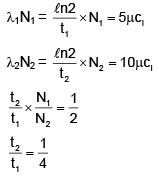Hence,
The half life of Sis 5 years, and
The half life of Sis 20 years

Q 34. The de-Broglie wavelength (λB) associated with the electron orbiting in the second excited state of hydrogen atom is related to that in the ground state (λG) by:    
(1) λB = 3λG
(2) λB = 2λG
(3) λB = 3λG/3
(4) λB = 3λG/2
Ans:
A
Solution: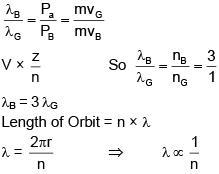Q 35.  Both the nucleus and the atom of some element are in their respective first excited states. They get de-excited by emitting photons of wavelengths λNA respectively. The ratio λNA  is closest to:     
(1) 10–1
(2) 10–6
(3) 10
(4) 10–10
Ans:
B
Solution: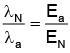where Ea and EN are energies of photons from atom and nucleus respectively. EN is of the order of MeV and Ea in few eV.
So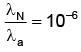Q 36. An electron beam is accelerated by a potential difference V to hit a metallic target to produce X-rays. It produces continuous as well as characteristic X-rays. If λmin is the smallest possible wavelength of X-ray in the spectrum, the variation of log λmin with log V is correctly represented in    
(1)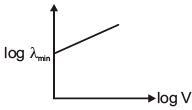(2)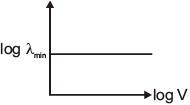(3)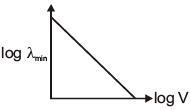(4)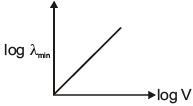Ans:
(3)
Solution:
In X-ray tube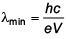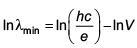Slope is negative
Intercept on y-axis is positive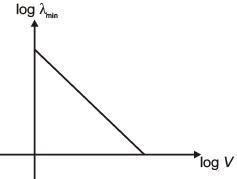Q 37. A particle A of mass m and initial velocity v collides with a particle B of mass m/2 which is at rest. The collision is head on, and elastic. The ratio of the de-Broglie wavelengths λA to λB after the collision is     
(1)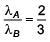(2)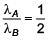(3)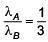(4)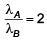Ans
: (4)
Solution: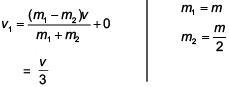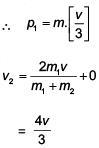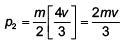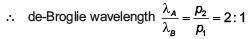Q 38. Some energy levels of a molecule are shown in the figure. The ratio of the wavelengths r = λ12, is given by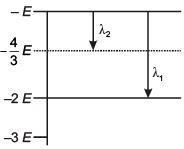(1)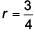(2)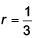(3)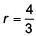(4)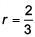Ans:
(2)
Solution:
From energy level diagram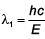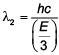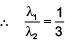Q 39. A radioactive nucleus A with a half life T, decays into a nucleus B. At t = 0, there is no nucleus B. At sometime t, the ratio of the number of B to that of A is 0.3. Then, t is given by    
(1) t =T log (1.3)
(2)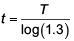(3)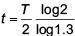(4)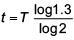Ans
: (4)
Solution: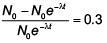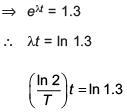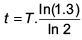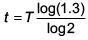Q 40.  In a certain region static electric and magnetic fields exist. The magnetic field is given by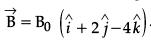.  If a test charge moving with a velocity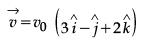experiences no force in that region, then the electric field in the region, in SI units, is:    
(1)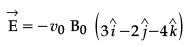(2)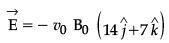(3)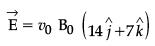(4)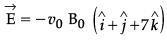Ans:
(2)
Solution:
Fe = Fn = 0
Fe = –Fm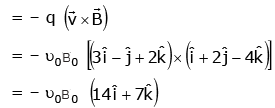Q 41.  What is the conductivity of a semiconductor sample having electron concentration of 5×1018 m−3, hole concentration of 5×1019 m−3, electron mobility of 2.0 m2 V−1 s−1 and hole mobility of 0.01 m2 V−1 s−1?
(Take charge of electron as 1.6×10−19 C)    
A: 0.59 (Ω - m)−1
B: 1.83 (Ω - m)−1
C: 1.68 (Ω - m)−1
D: 1.20 (Ω - m)−1
Ans:
(3)
Solution:
s = e (neμe + nnμn)
= 1.6 × 10-19 (5 × 1018 × 2 + 5 × 1019 × 0.01)
= 1.6 × 10–19 (1019  + 0.05 × 1019)
= 1.6 × 1.05
= 1.68

Q 42.  A signal of frequency 20 kHz and peak voltage of 5 Volt is used to modulate a carrier wave of frequency 1.2 MHz and peak voltage 25 Volts. Choose the correct statement.    
(1) Modulation index = 5, side frequency bands are at 1400 kHz and 1000 kHz
(2) Modulation index = 5, side frequency bands are at 21.2 kHz and 18.8 kHz
(3) Modulation index = 0.8, side frequency bands are at 1180 kHz and 1220 kHz
(4) Modulation index = 0.2, side frequency bands are at 1220 kHz and 1180 kHz
Ans:
(4)
Solution: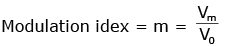=1/5 = 0.2
Frequency = 12 × 103 kHz
F = 12.00 kHz
F1 = 1200 – 20 = 1180 kHz
F2 = 1200 + 20 = 1220 kHz

Q 43. A negative test charge is moving near a long straight wire carrying a current. The force acting on the test charge is parallel to the direction of the current. The motion of the charge is:    
(1) away from the wire
(2) towards the wire
(3) parallel to the wire along the current
(4) parallel to the wire opposite to the current
Ans:
(1)
Solution: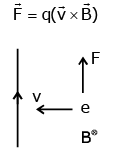Q 44.  A single slit of width 0.1 mm is illuminated by a parallel beam of light of wavelength 6000 Å and diffraction bands are observed on a screen 0.5 m from the slit. The distance of the third dark band from the central bright band is:    
(1) 3 mm
(2) 9 mm
(3) 4.5 mm
(4) 1.5 mm
Ans:
(2)
Solution:
a = 0.1 mm = 10–4
λ = 6000 × 10-10
= 6 × 10-7
D = 0.5 m
for 3rd dark
a sin θ = 3λ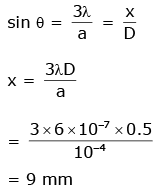Q 45. Radiation of wavelength λ, is incident on a photocell. The fastest emitted electron has speed v. If the wavelength is changed to 3λ/4, the speed of the fastest emitted electron will be:    
(1)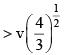(2)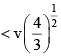(3)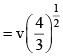(4)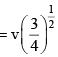Ans:
A
Solution: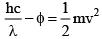.... (i)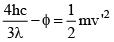...... (ii)
(ii) - (i) gives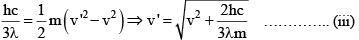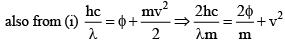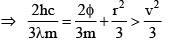.... (iv)
combining (iii) & (iv)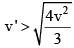Q 46. Half-lives of two radioactive elements A and B are 20 minutes and 40 minutes, respectively. Initially, the samples have equal number of nuclei. After 80 minutes, the ratio of decayed numbers of A and B nuclei will be:    
(1) 1 : 16
(2) 4 : 1
(3) 1 : 4
(4) 5 : 4
Ans:
D
Solution:
80 minutes = 4 half-lives of A = 2 half-lives of B
Let the initial number of nuclei in each sample be N
NA after 80 minutes = N/24
⇒ Number of A nuclides decayed = 15/16N
NB after 80 minutes = N/22
⇒ Number of B nuclides decayed = 3/4N
Required ratio =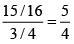Q 47. A hydrogen atom makes a transition from n = 2 to n=1 and emits a photon. This photon strikes a doubly ionized lithium atom (z = 3) in excited state and completely removes the orbiting electron. The least quantum number for the excited states of the ion for the process is:     
(1) 4
(2) 5
(3) 2
(4) 3
Ans:
(1)
Solution:
Energy of proton = 13.6 – 3.4 = 10.2 eV
For removal of electron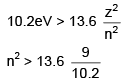so minimum value of n = 4

Q 48. When photons of wavelength λ1, are incident on an isolated sphere, the corresponding stopping potential is found to be V. When photons of wavelength λ2, are used , the corresponding stopping potential was thrice that of the above value. If light of wavelength λ3. is used then find the stopping potential for this case     
(1)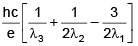(2)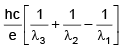(3)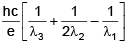(4)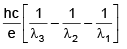Ans:
(1)
Solution: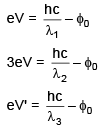using these equations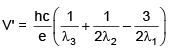Q 49. Microwave oven acts on the principle of:     
(1) giving rotational energy to water molecules
(2) giving vibrational energy to water molecules
(3) giving translational energy to water molecules
(4) transferring electrons from lower to higher energy levels in water molecule
Ans.
(2)
Solution: Energy of microwaves lie in range of vibration energy of water molecules.

Q 50. A neutron moving with a speed 'v' makes a head on collision with a stationary hydrogen atom in ground state. The minimum kinetic energy of the neutron for which inelastic collision will take place is:    
(1) 10.2 eV
(2) 12.1 eV
(3) 20.4 eV
(4) 16.8eV
Ans:
(3)
Solution: Assuming perfectly inelastic collision for maximum loss in K.E.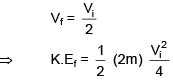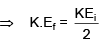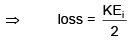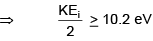⇒ K.Ei ≥ 20.4 eV

Q 51. A photoelectric surface is illuminated successively by monochromatic light of wavelengths λ and λ/3.  If the maximum kinetic energy of the emitted photoelectrons in the second case is 3 time that in the first case, the work function of the surface is:   
(1) 3hc/λ
(2) hc/λ
(3) hc/3λ
(4) hc/2λ
Ans:
(4)
Solution: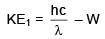...(1)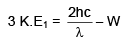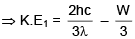...(2)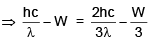(Equating (i) & (ii))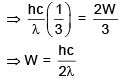Offer running on EduRev: Apply code STAYHOME200 to get INR 200 off on our premium plan EduRev Infinity!

42 docs|19 tests

,

,

,

,

,

,

,

,

,

,

,

,

,

,

,

,

,

,

,

,

,

;